## Angular Momentum

For the common problem of central potentials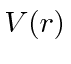, we use the obvious rotational symmetry to find that the angular momentum,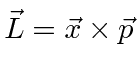, operators commute with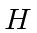,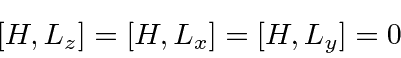but they do not commute with each other.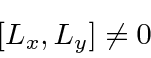We want to find two mutually commuting operators which commute with, so we turn towhich does commute with each component of.We chose our two operators to be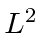and.

Some computation reveals that we can writeWith this the kinetic energy part of our equation will only have derivatives inassuming that we have eigenstates of.The Schrödinger equation thus separates into an angular part (theterm) and a radial part (the rest). With this separation we expect (anticipating the angular solution a bit)will be a solution. The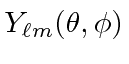will be eigenfunctions ofso the radial equation becomesWe must come back to this equation for eachwhich we want to solve.

We solve the angular part of the problem in general using angular momentum operators. We find that angular momentum is quantized.withandintegers satisfying the condition. The operators that raise and lower the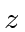component of angular momentum areWe derive the functional form of the Spherical Harmonicsusing the differential form of the angular momentum operators.

Jim Branson 2013-04-22